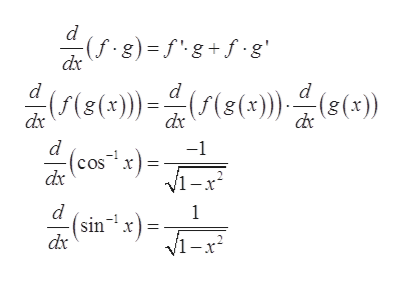Question
23 views

Find the derivative

f(x) = cos-1(3x) sin-1(3x)

check_circle

Step 1

To differentiate the function with respect to x.

Step 2

Given:

Step 3

Formula Used:

...help_outlineImage Transcriptionclosed (f. g) = f'g +f.g' dx d ()e(e) d (f(8(x)) dix (8(x) :(5(g(x)))- dx d -1 (cosx) = dx V1-x2 1 (sin x) = Vi-x dx fullscreen

### Want to see the full answer?

See Solution

#### Want to see this answer and more?

Solutions are written by subject experts who are available 24/7. Questions are typically answered within 1 hour.*

See Solution
*Response times may vary by subject and question.
Tagged in

### Derivative